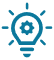In this course we will see how to use mathematical functions in your program.In fact, it can be really useful to calculate the square root of a number, or having it’s absolute value.

## Arithmetic operation

This is a list of basic operation:

Operator Code
Addition : + int addition =5 + 5 // Return 10 into the variable
Subtraction : – int subtracting =5 – 5 // Return 0 into the variable
Multiplication : * int multiplication = 7*2; // return 14 into the variable
Divison : / int division = 6/2; // return 3 in the variable
Modulo : % int modulo =7 % 5 // return 2 in the variable

## Comparison operator

Operator Code
Is to not equal to : != if (5 !=8) { // Your code }; //Here we enter in the loop because 5 is different to 8.
Smaller than: < if (2<3) { // Your code }; // We enter in the loop because 2 is smaller than 3.
Smaller or equal to : <= if (2<=2) { // Your code }; //Here we enter in the loop because 2 is smaller or equal to 3.
Equal to : == if (6==6) { // Your code }; // Here we enter in the loop because 6 is equals to 6.
Bigger than : > if (9>1) { // Your code }; // Here we enter in the loop because 9 is bigger than 1
Bigger or equal to : >= if (9>=9) { // Your code }; // Here we enter in the loop because 9 is bigger or equal to 9.

## Boolean operator

Opérateur Code
Logical NOT : ! if (!x) { //  if x is False Your code }
Logical AND : && if (5==5 && 2==2) { // The both conditions are true therefore we enter in the code. }
Logical OR : || if (3==3 || 4==4) { // We enter in this code because one of the two equalities is true. };

## Absolute function: abs()

The function absolute value is used to be sure that a number is positive or null. This function can be also used to transform a negative number to a positive one.

The abs() function take in parameter a number positive or negative and return the same number in positive:

``abs(number);``
Here is an example with a negative number -5:
``abs(-5); // return 5``
By taking 0, the function return 0:
``abs(0); // return 0``

## Maximum function: max()

The maximum function take in parameters two numbers and return the biggest of the two :
``max(x,y);``
Example between two numbers: x=23 and y=2 :
``max(23,2);// Return 23``

The function takes in parameter any type of variable: string, int, float…### The maximum function can be also used to have a minimum value!

In your program, you may need to retrieve a value of a sensor or have a minimum value in case if the value of the sensor is to low.

Here is an example:

``value_sensor =max(photoresistor, 20)// Take the value of the photoresistor if this value is bigger than 20 ``

In this example, the variable value_sensor take the value of the photoresistor if this the value is bigger than 20, otherwise it will take 20.

## Minimum function: min()

The minimum function is the reverse of the maximum function, that ‘s mean that the function takes two values and return the smallest one.

``min(x,y);``

Here is an example with the numbers : x=23, y=2:

``min(23,2);// Return 2``

The function return 2. X and Y can have a different type: string, int, float…### The maximum function can be also used to have a minimum value!

In a program, you can also need to retrieve a value from a sensor or a maximum value in case if the value of the sensor is too high.

Here is an example:

``value_sensor =min(photoresistor, 20)// Take the value of the photoresistor if inferior to 20``

In this example, the variable value_sensor takes the value of the photoresistor if it’s lower than 20, otherwise it will take 20.

## Square root function: sqrt()

The function sqrt is used to have the square root of a number:

``sqrt(x) // Square root of the number x``

The function sqrt() return a double.

Here is an example with the number 25:

``````sqrt(25) // The square root of 25 return 5.00
``````

## Trigonometry : cosine, sine and tangent

We will see now how the cosine, sine and tangent works:

• Cosine

The cosine function takes in parameter an angle in radian and return a value between 1 and -1:

``cos(rad); // return a value between 1 and -1 ``

The function takes in parameter a float and return a double.

Here is an example with the cosine with an angle of 90°:

``cos(90); // return the value -0.45``
• Sine

The sine function takes in parameter an angle in radian and return a value between 1 to -1:

``sin(rad); // return the value between 1 and -1. ``

The function takes in parameter a float and return a double.

Here is an example with the sine with an angle of 90°:

``sin(90); // return the value 0.89``
• Tangent

The function tangent takes in parameter an angle in radian and return a value between minus infinite to infinite:

``````tan(rad); // return a value between minus infinite and infinite
``````
• Here is an example with the value 0:
``tan(0); // return 0.00``